# 4 Session 4: Model Analysis

library(daymetr)
library(dplyr)
library(ggplot2)
theme_set(theme_bw())
options(digits = 3)

## 4.1 Learning Objectives

Today you will learn to

• Use visual and statistical approaches to compare model output to data
• Investigate the relationships between model inputs and outputs using sensitivity analysis and variance decomposition

## 4.2 Comparing models to data

DayMet is a gridded weather data product. It provides daily weather statistics for North America on a 1 km grid. The primary input to DayMet is data from weather stations. Using a number of statistical methods, values for each square on the grid are imputed.

Today we are going to use these as our ‘model,’ and we are going to compare the output of this model to data from a weather station in Maricopa, AZ.

Lets download the daily weather data from DayMet:

mac_daymet_list <- download_daymet(site = "Maricopa Agricultural Center",
lat = 33.07,
lon =  -111.97,
start = 2020,
end = 2020,
internal = TRUE) 
## Downloading DAYMET data for: Maricopa Agricultural Center at 33.07/-111.97 latitude/longitude !
## Done !
obs <- comparison$tmean_obs reg <- lm(obs~ mod) plot(mod, obs, xlab = 'model tmean', ylab = 'obs tmean') + abline(0,1) + abline(coef(reg), lty = 2)## integer(0) summary(reg) ## ## Call: ## lm(formula = obs ~ mod) ## ## Residuals: ## Min 1Q Median 3Q Max ## -10.24 -2.85 -0.34 2.43 10.12 ## ## Coefficients: ## Estimate Std. Error t value Pr(>|t|) ## (Intercept) 2.1546 0.7042 3.06 0.0024 ** ## mod 0.8514 0.0263 32.39 <2e-16 *** ## --- ## Signif. codes: ## 0 '***' 0.001 '**' 0.01 '*' 0.05 '.' 0.1 ' ' 1 ## ## Residual standard error: 3.95 on 309 degrees of freedom ## Multiple R-squared: 0.772, Adjusted R-squared: 0.772 ## F-statistic: 1.05e+03 on 1 and 309 DF, p-value: <2e-16 Does the regression model includes the 1:1 line? Why is the P-value so low? because the default hypothesis test is that the slope is different than 0, not different than 1 coef(reg) ## (Intercept) mod ## 2.155 0.851 confint(reg) ## 2.5 % 97.5 % ## (Intercept) 0.769 3.540 ## mod 0.800 0.903 Is the slope 1:1? What about for tmin and tmax? summary(lm(tmin_obs ~ tmin_model, data = comparison)) summary(lm(tmax_obs ~ tmax_model, data = comparison)) #### 4.2.1.2 RMSE Root Mean Square Error This is the most common model metric. It is the standard deviation of the residuals. $RMSE=\sqrt{\frac{1}{n}(Y_{model}-Y_{obs})^2}$ RMSE <- sqrt(mean((mod - obs)^2)) RMSE  ##  4.45 Sometimes you want a “Normalized” estimate of model error. That way the magnitude of the error is on the same scale as the observations. There are many ways to do this, a common approach is to divide by the mean of the observations. $$NRMSE=RMSE/\bar{Y}_{obs}$$ is equivalent to coefficient of variance $$CV=\frac{\sigma}{\bar{x}}$$. ## to normalize, divide by sd(obs) NRMSE <- RMSE/sd(obs) Other approaches to normalization: • $$RMSE/sd(obs)$$ • $$RMSE/\bar{Y_{obs}}$$ • $$RMSE/IQR$$ #### 4.2.1.3$$R^2$$ Corellation Coefficient When comparing models to data, it is common to calculate the Correlation Coefficient in terms of deviation from the 1:1 line $R^2=1-\frac{\sum{(Y_{mod}-Y_{obs})^2}}{\sum{(Y_{obs}-\bar{Y}_{obs})^2}}$ cor(mod, obs) ##  0.879 cor.test(mod, obs) ## ## Pearson's product-moment correlation ## ## data: mod and obs ## t = 32, df = 309, p-value <2e-16 ## alternative hypothesis: true correlation is not equal to 0 ## 95 percent confidence interval: ## 0.851 0.902 ## sample estimates: ## cor ## 0.879 #### 4.2.1.4 Bias Do you over or under predict? $Y_{model}-Y_{obs}$ mean(mod - obs) ##  1.62 # normalized mean(mod - obs)/mean(obs) ##  0.068 It is also useful to visualize and compute statistics on these differences. hist(mod-obs)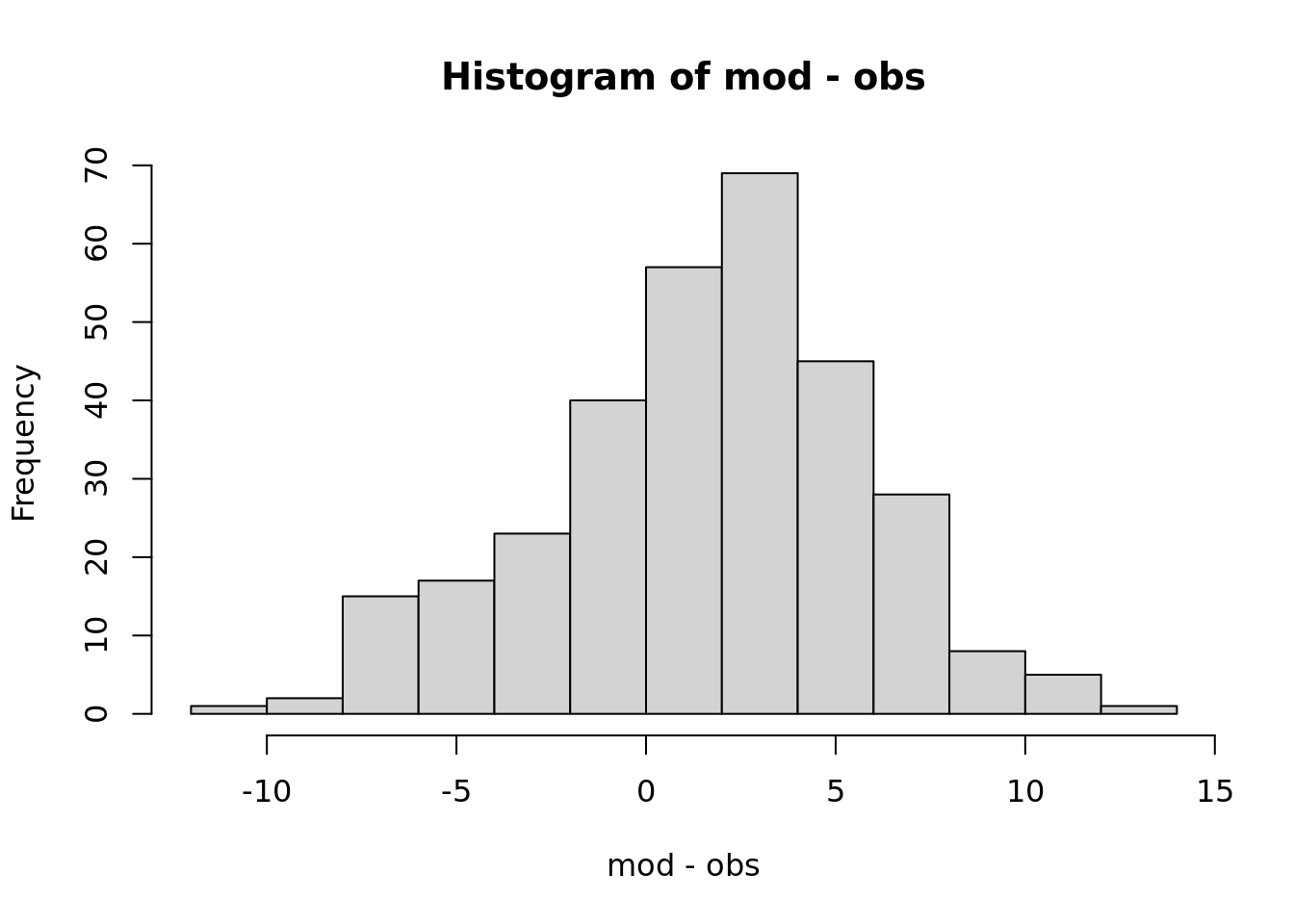What fraction of the time is the model above the observation? sum(mod > obs) / length(obs) ##  0.685 #### 4.2.1.5 Ratio of variances Does the model capture the observed variance? Often a model will have lower variances … why? $\frac{SD_{Y_{model}}}{SD_{Y_{obs}}}$ sd(obs) / sd(mod) ##  0.969 #### 4.2.1.6 Taylor Diagram - three metrics in two dimensions A Taylor Diagram allows you to plot three metrics of model performance - the RMSE, R^2, and SD ratio in two dimensions, taking advantage of the mathematical relationships among these scores. It is common to plot multiple points representing different models or model parameterizations, also different years or locations that the model is evaluated at. Plotting these as normalized values allows comparison across variables being predicted. library(plotrix) taylor.diagram(ref=obs, model = mod, normalize = TRUE, ref.sd = TRUE)## 4.3 Simulation Models Yesterday I introduced the concept and application of simulation models, with examples related to simulating plant growth. In a regression model, it is often possible to see how each parameter relates to the model output (e.g. slope, intercept). For a complex model, it is often not possible to understand the high level dynamics of the system by analyzing the mathematics. Another approach is to treat the model as a ‘black box.’ Then we can treat the black box as an experimental system, purturb it and see how it responds. Lets look at this in more detail. ### 4.3.1 Example: A leaf level model of photosynthesis Based on the coupled C4 photosynthesis - conductance model developed by Collatz and Ball Berry G. Collatz, M. Ribas-Carbo, J. Berry. (1992). Coupled photosynthesis-stomatal conductance model for leaves of C4 plants. Australian Journal of Plant Physiology 519–538. Water transpiration is coupled to photosynthesis through stomatal conductance, since plants need to regulate water loss as they uptake $$CO_2$$: $g_s = m\frac{A_n h_s}{c_a}p + b$ Where $$g_s$$ is stomatal conducatnce, $$A_n$$ is net photosynthesis, $$h_s$$ is relative humidity, $$c_a$$ is $$CO_2$$ at leaf surface. and Photosynthesis is $A_n=min(A_c, A_L)-R_d$ Where Rubisco-limited rate is $$A_c$$ and RuBP limited rate is $$A_L$$ $A_c=V_m\left[\frac{c_i-\Gamma}{c_i+K_c(1+O_2/K_o)}\right]$ $A_L=$ This is a non-linear equation with key plant physiological traits: Parameter Description Vmax maximum carboxylation of Rubisco according to the Collatz model. alpha alpha parameter according to the Collatz model. Initial slope of the response to Irradiance. kparm k parameter according to the Collatz model. Initial slope of the response to CO2. theta theta parameter according to the Collatz model. Curvature for light response. beta beta parameter according to the Collatz model. Curvature for response to CO2. Rd Rd parameter according to the Collatz model. Dark respiration. b0 intercept for the Ball-Berry stomatal conductance model. b1 slope for the Ball-Berry stomatal conductance model. The rate of photosynthesis is determined by environmental factors: Parameter Description Tl temperature of the leaf (Celsius). RH relative humidity (as a fraction, i.e. 0-1). Qp quantum flux (direct light), (micro mol m-2 s-1). Catm Atmospheric CO2 in ppm (or micromol/mol). In the end: $[Gs, A, C_i]=f(T, RH, Light, CO_2, v_{max}, \alpha, k, \theta, R_d, b_0, b_1)$ ### 4.3.2 Let’s run this model! First, lets load some meteorological data: library(BioCro) #devtools::install_github('ebimodeling/biocro') library(lubridate) library(ggplot2) data("weather04") time <- ymd('2004-01-01') + days(weather04$doy-1) + hours(weather04$hour) par <- weather04$solarR
rh <- weather04$RH temp <- weather04$DailyTemp.C

Now, estimate photosynthesis rate at each time step. This is isn’t the entire model - just what would be happening to the leaf of a plant with C4 photosynthesis in full sunlight.

A <- c4photo(Qp = par, Tl = temp, RH = rh)$Assim pairs(data.frame(A, par, temp, rh))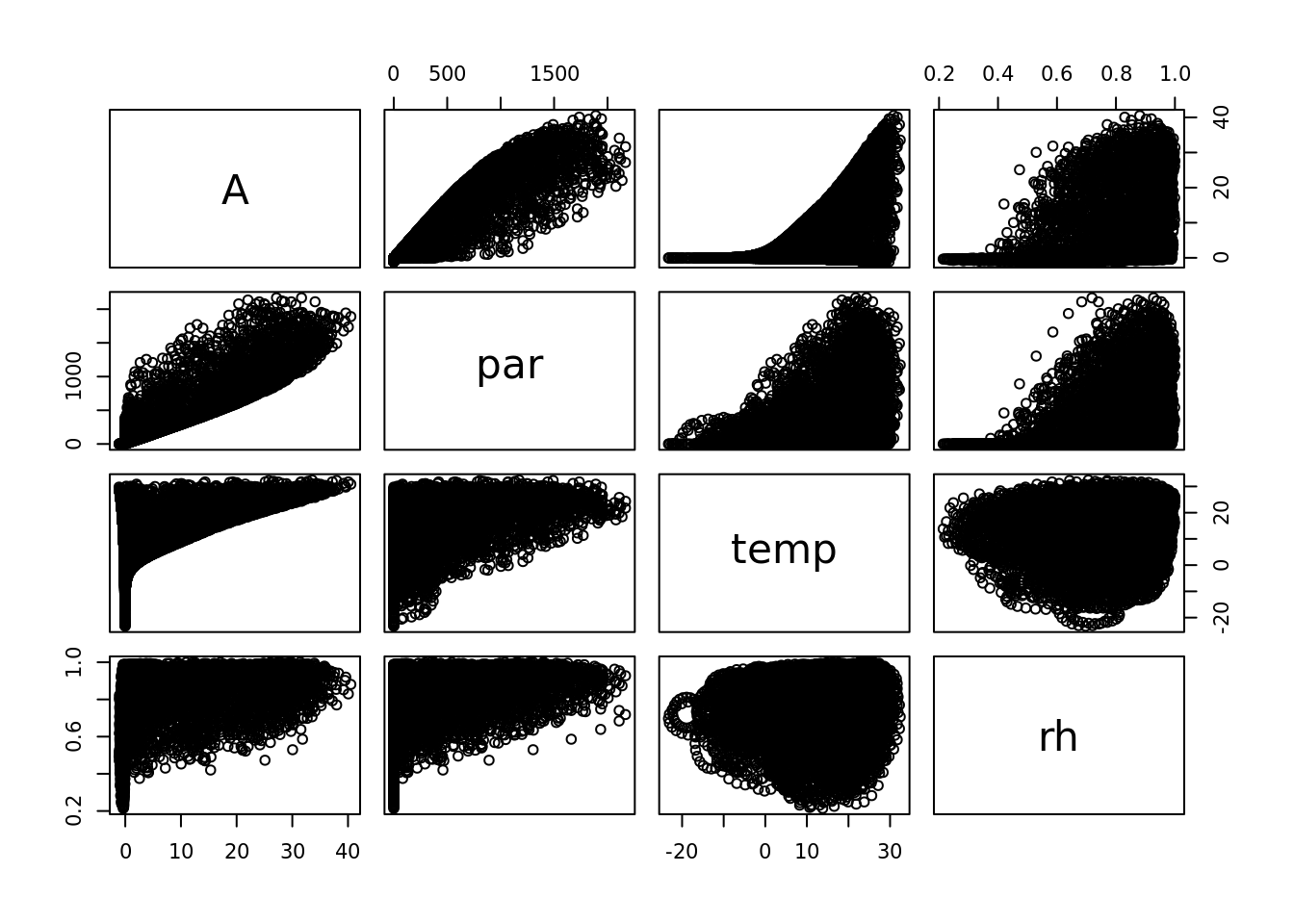## whoa this is wierd! What is going on? plot(temp, rh)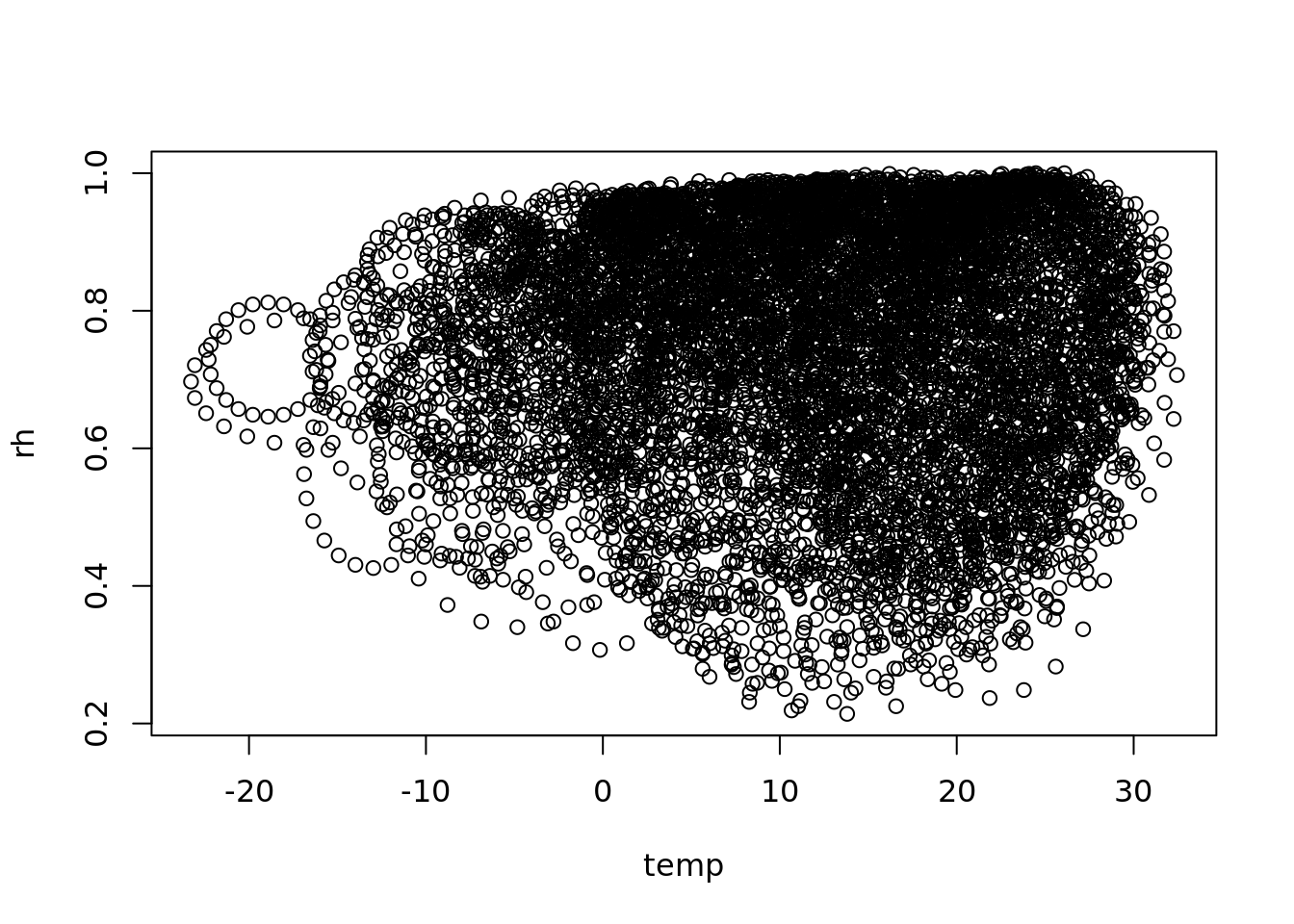a strange scaling algorithm, interpolating from daily to hourly see also https://rpubs.com/dlebauer/metdriver_comparisons library(ggplot2) ggplot()+ geom_line(aes(time, A)) + scale_x_datetime(limits = ymd_h(c('2004-05-01 0', '2004-06-01 23'))) ## Warning: Removed 7992 row(s) containing missing values ## (geom_path).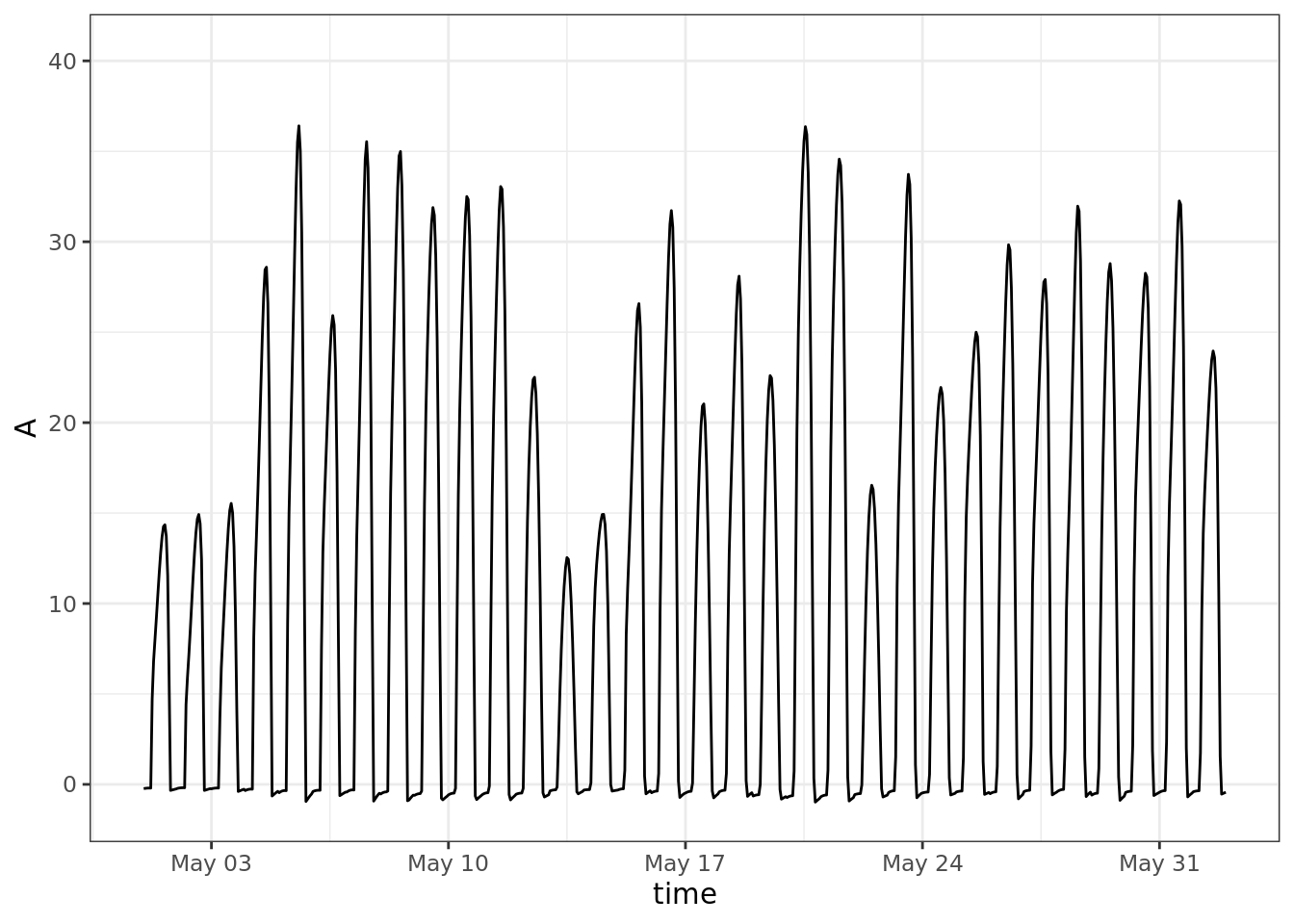ggplot()+ geom_line(aes(time, rh)) + scale_x_datetime(limits = ymd_h(c('2004-05-01 0', '2004-06-01 23'))) ## Warning: Removed 7992 row(s) containing missing values ## (geom_path).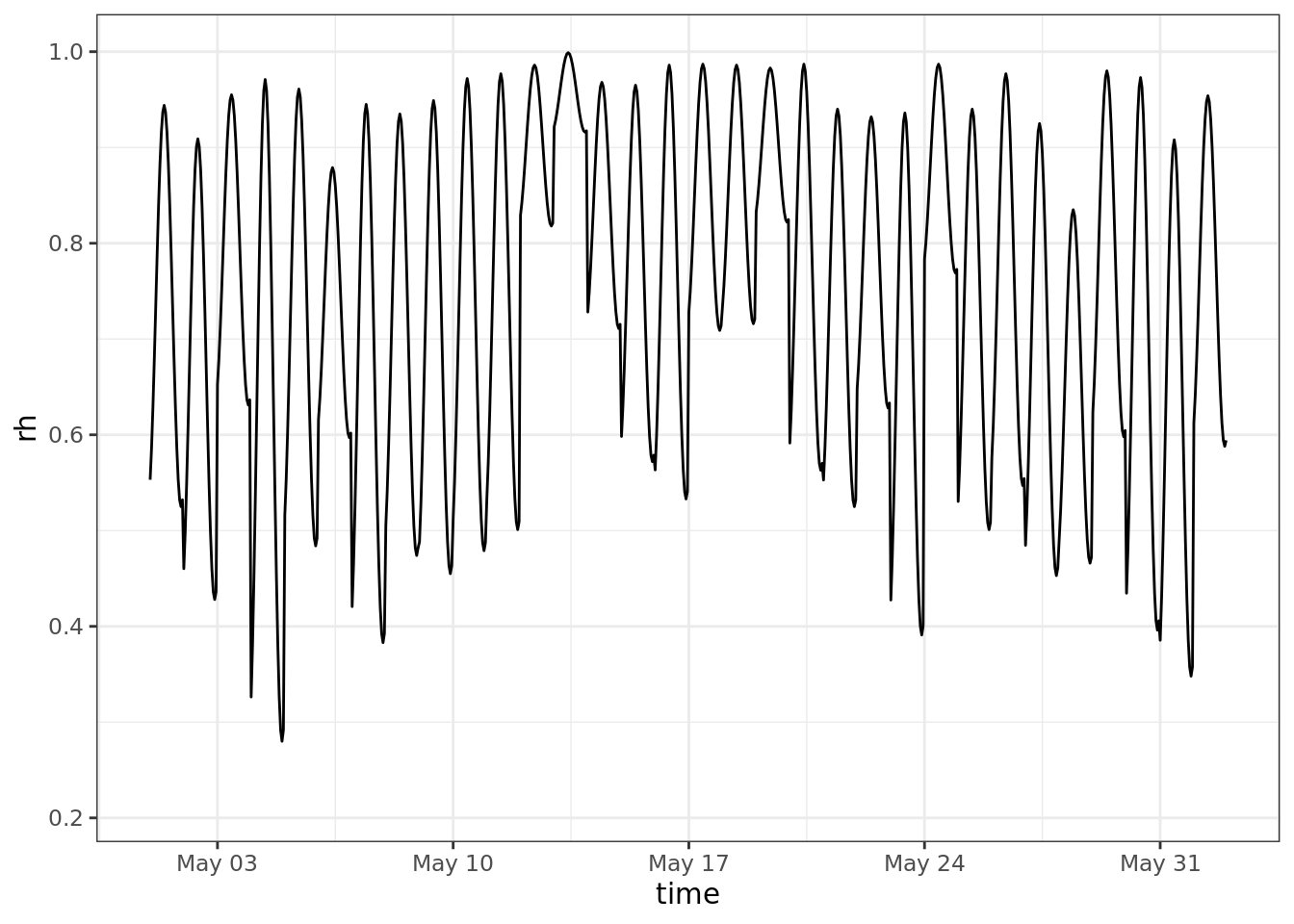question: is f(mean(X)) = mean(f(X))? testQp <- 11:20*100 testTl <- 21:30 testRH <- 21:30/50 A1 <- c4photo(Qp = mean(testQp), Tl = mean(testTl), RH = mean(testRH)) A2 <- lapply(c4photo(Qp = testQp, Tl = testTl, RH = testRH), mean) dplyr::bind_rows(A1 = A1, A2 = A2) ## # A tibble: 2 × 4 ## Gs Assim Ci GrossAssim ## <dbl> <dbl> <dbl> <dbl> ## 1 239. 31.3 171. 32.1 ## 2 243. 31.4 173. 32.2 Why are these different? For non-linear functions see Jensen’s Inequality (Wikipedia) This means - be careful when and how you use averages - everywhere!!! Spatial and temporal downscaling is how crop modelers deal with lower resolution atmospheric model forecasts. For example the most recent IPCC 100 y climate forecasts were generated on ~100km grids (Taylor et al 2012), thus one data point may simultaneously represent a month that is $$60^o$$F and foggy in San Fransicsco and $$100^o$$F and dry in Davis, CA. At the same time, crop models need to run on local hourly data while also capturing the uncertainty represented by within and across model variability. #### 4.3.2.1 Model sensitivity Next, we are going to look at the sensitivity of the model to each of the different weather parameters, holding the others at their mean meanQp <- mean(par) meanTl <- mean(temp) meanRH <- mean(rh) plot(1:100/100, c4photo(Qp = rep(meanQp, 100), Tl = rep(meanTl, 100), RH = 1:100/100)$Assim,
type = 'l', ylab = 'Assim', xlab = 'RH')plot(1:100/4, c4photo(Qp = rep(meanQp, 100),
Tl = 1:100/4,
RH = rep(meanRH, 100))$Assim, type = 'l', ylab = 'Assim', xlab = 'RH')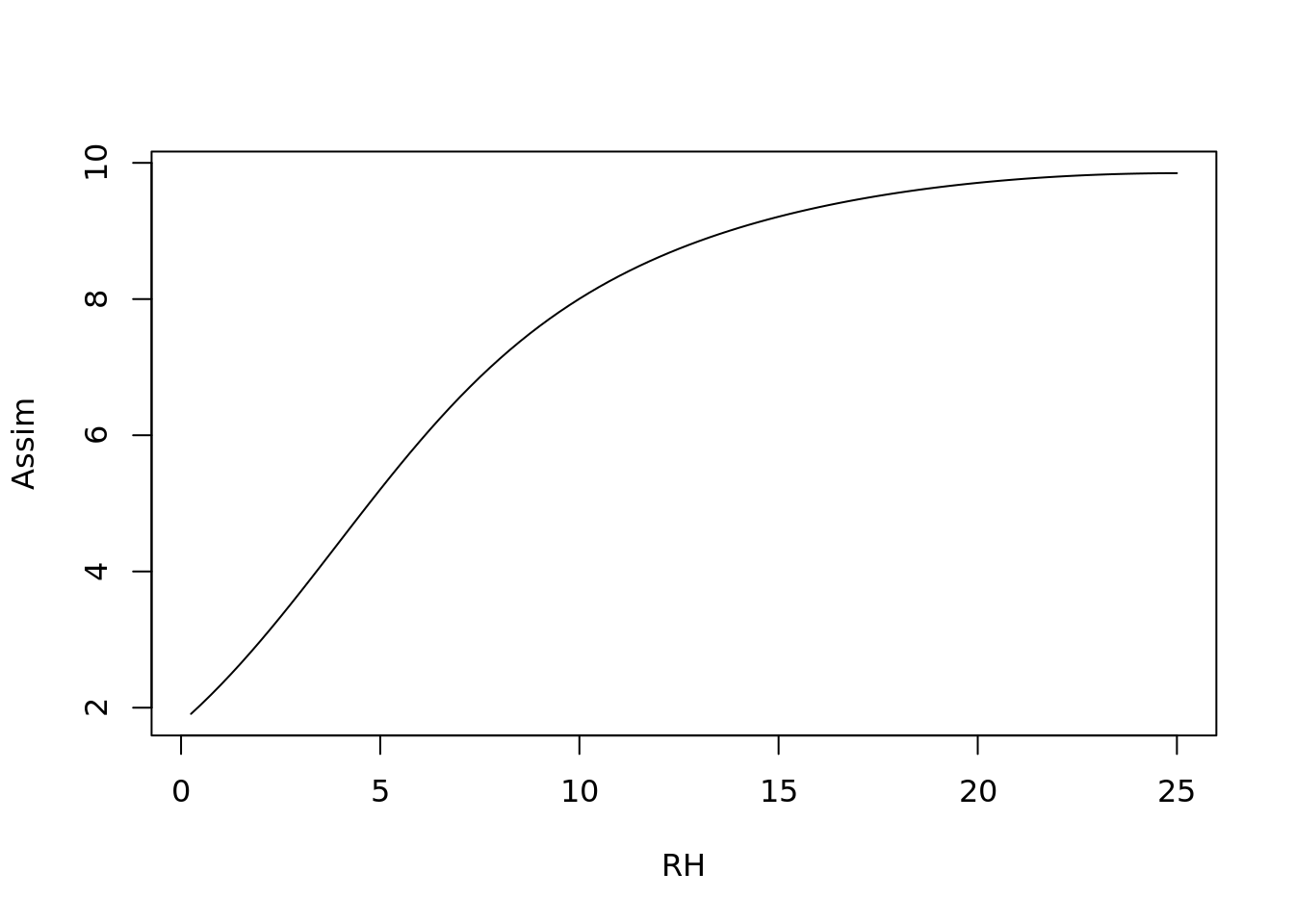#### 4.3.2.2 Monte Carlo Error propagation Given a model parameters represented as variables, e.g. $V_{cmax}\sim N(45,2)\\ Rd\sim N(1, 0.1) b1 \sim N(4,1)$ What is expected uncertainty in model predictions of photosynthesis (A = assimilation) Lets take some samples from these distributions set.seed(100) n <- 1000 vmax <- rnorm(n, 45, 2) Rd <- rnorm(n, 1, 0.10) b1 <- rnorm(n, 4, 1) Now we can take a look at them x <- 25:75 ggplot() + geom_histogram(aes(vmax, y = ..density..), proability = TRUE) + geom_line(aes(x, dnorm(x, 45, 2))) ## Warning: Ignoring unknown parameters: proability ## stat_bin() using bins = 30. Pick better value with ## binwidth.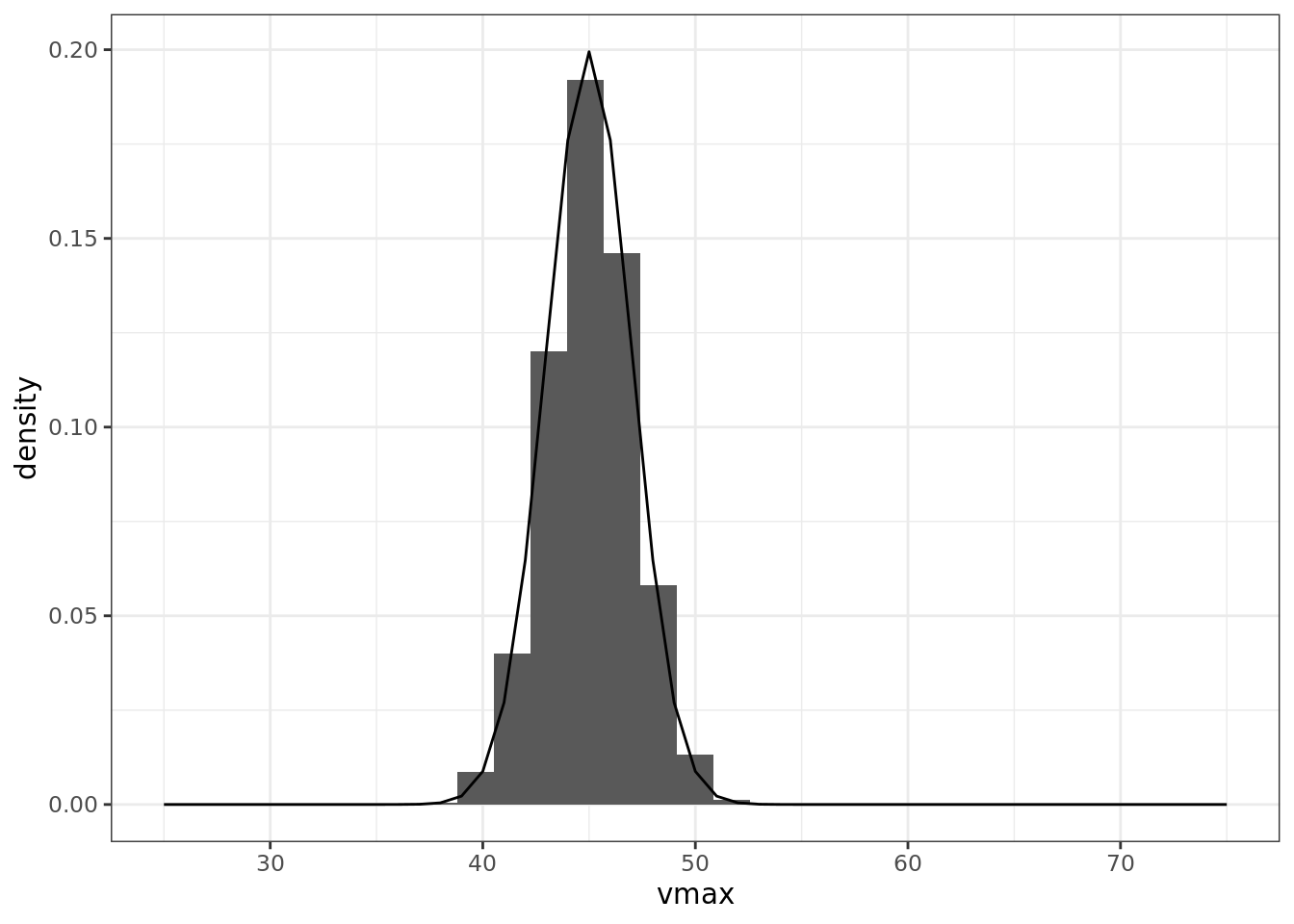x <- 1:200/100 ggplot() + geom_histogram(aes(Rd, y = ..density..), proability = TRUE) + geom_line(aes(x, dnorm(x, 1, 0.1))) ## Warning: Ignoring unknown parameters: proability ## stat_bin() using bins = 30. Pick better value with ## binwidth.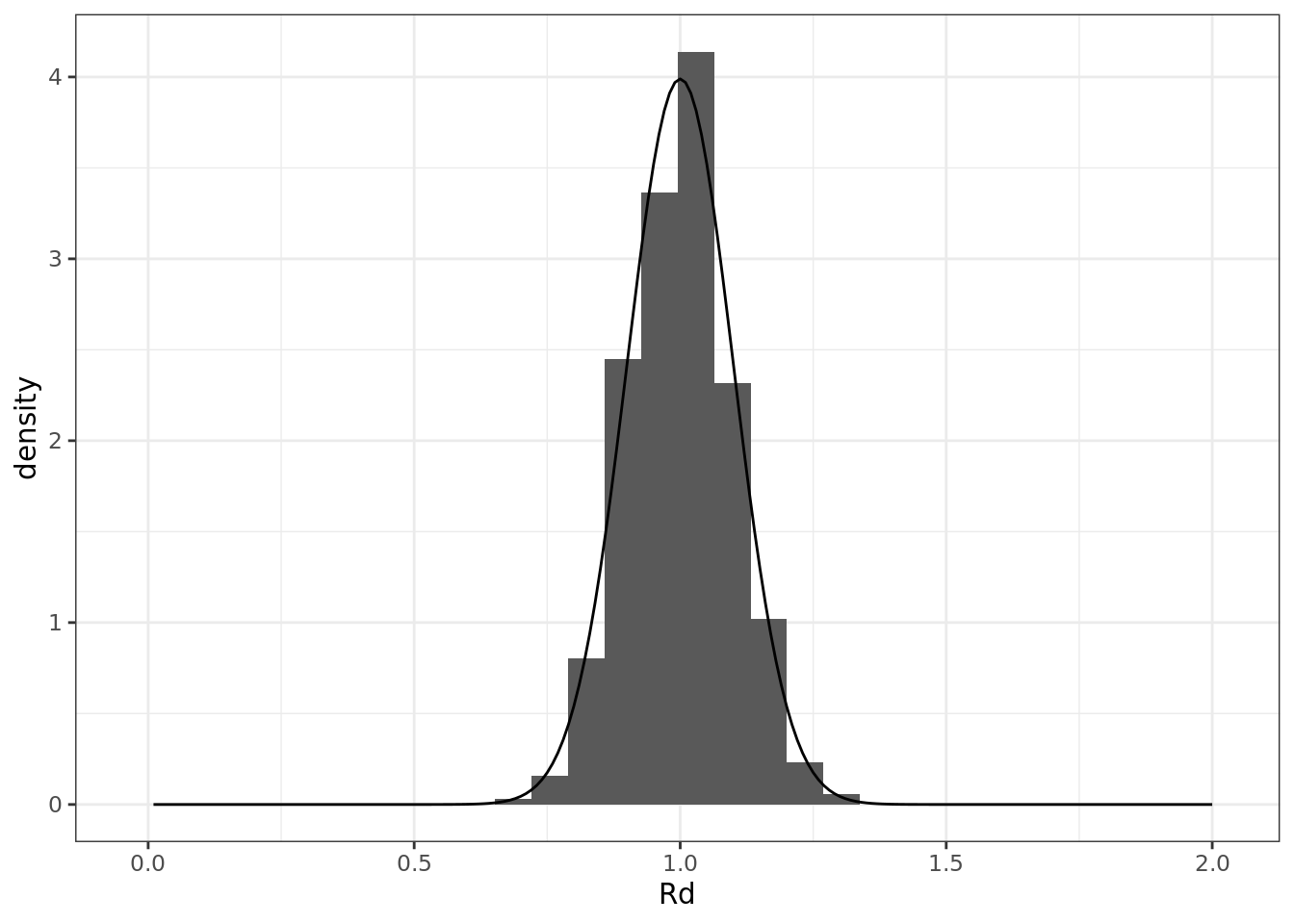Next, we are going to propagate the parameter variance to see how much of an effect it has on the model output variance: ### sample given time series of met A <- matrix(nrow = length(time), ncol = 1000) for(i in 1:1000){ A[,i] <- c4photo(Qp = par, Tl = temp, RH = rh, vmax = vmax[i], Rd = Rd[i], b1=b1[i])$Assim
}

# take a look at the matrix ..
# image(A, xlab = 'time', ylab = 'sample')
## shows an annual cycle of photosynthesis
median <- which.min(abs(quantile(colMeans(A), 0.50)-colMeans(A)))
ucl    <- which.min(abs(quantile(colMeans(A), 0.975)-colMeans(A)))
lcl    <- which.min(abs(quantile(colMeans(A), 0.025)-colMeans(A)))

ggplot() +
#  geom_smooth(aes(time, A))+
geom_line(aes(time, A[,median])) +
geom_line(aes(time, y = A[,lcl]), linetype = 2) +
geom_line(aes(time, y = A[,ucl]), linetype = 2) +
scale_x_datetime(limits = ymd_h(c('2004-05-01 0', '2004-05-07 23')))
## Warning: Removed 8592 row(s) containing missing values
## (geom_path).

## Warning: Removed 8592 row(s) containing missing values
## (geom_path).

## Warning: Removed 8592 row(s) containing missing values
## (geom_path).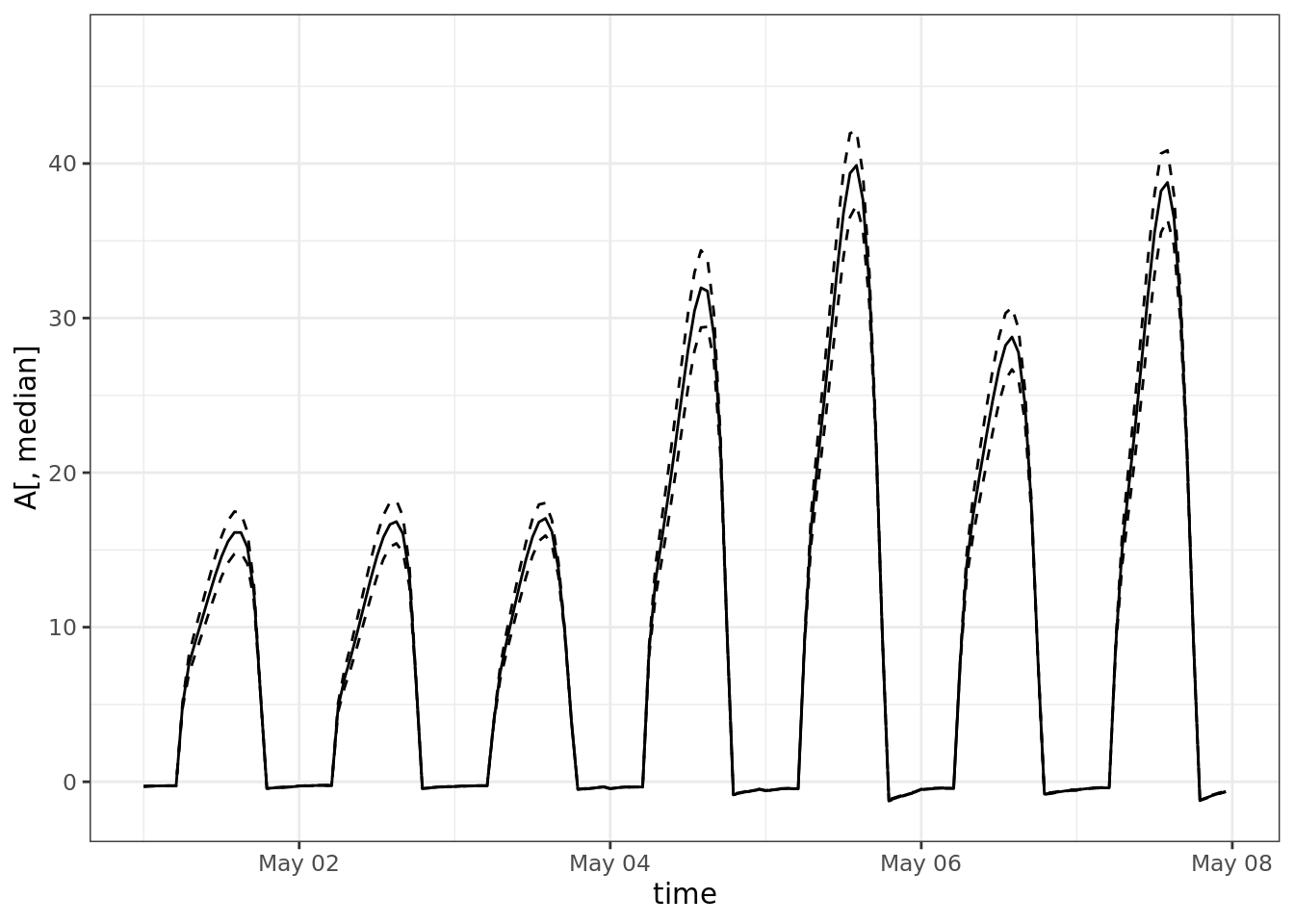### 4.3.3 Variance Decomposition - which of these parameters are most important?

Response of interest is the total assimilation

a_total <- colMeans(A)

summary(aov(a_total ~ vmax + Rd + b1))
##              Df Sum Sq Mean Sq F value Pr(>F)
## vmax          1  22.66   22.66    8593 <2e-16 ***
## Rd            1   2.55    2.55     966 <2e-16 ***
## b1            1   0.40    0.40     153 <2e-16 ***
## Residuals   996   2.63    0.00
## ---
## Signif. codes:
## 0 '***' 0.001 '**' 0.01 '*' 0.05 '.' 0.1 ' ' 1

### 4.3.4 Propagate met and Use met as a variable, sample over variation within the hour

To answer the question - if I were to step out and look at the plants in the summer, how fast would they be growing?

This time, instead of sampling from a parameterized distribution, I am going to sample over the middle (hours 10-4) of days in the middle of the year (approx. summer, day of year 120-240)

### sample over met variability

A2 <- Gs <- Ci <- Qp <- Tl <- RH <- vector(length = 1000)
for(i in 1:1000){
day <- sample(120:240, size = 1)
hour <- sample(10:16, size = 1)
j <- day * 24 + hour
Qp[i] <- par[j]
Tl[i] <- temp[j]
RH[i] <- rh[j]
res <- c4photo(Qp = Qp[i], Tl = Tl[i], RH = RH[i], vmax = vmax[i], Rd = Rd[i], b1=b1[i])
A2[i] <- res$Assim Gs[i] <- res$Gs
Ci[i] <- res\$Ci
}

hist(A2)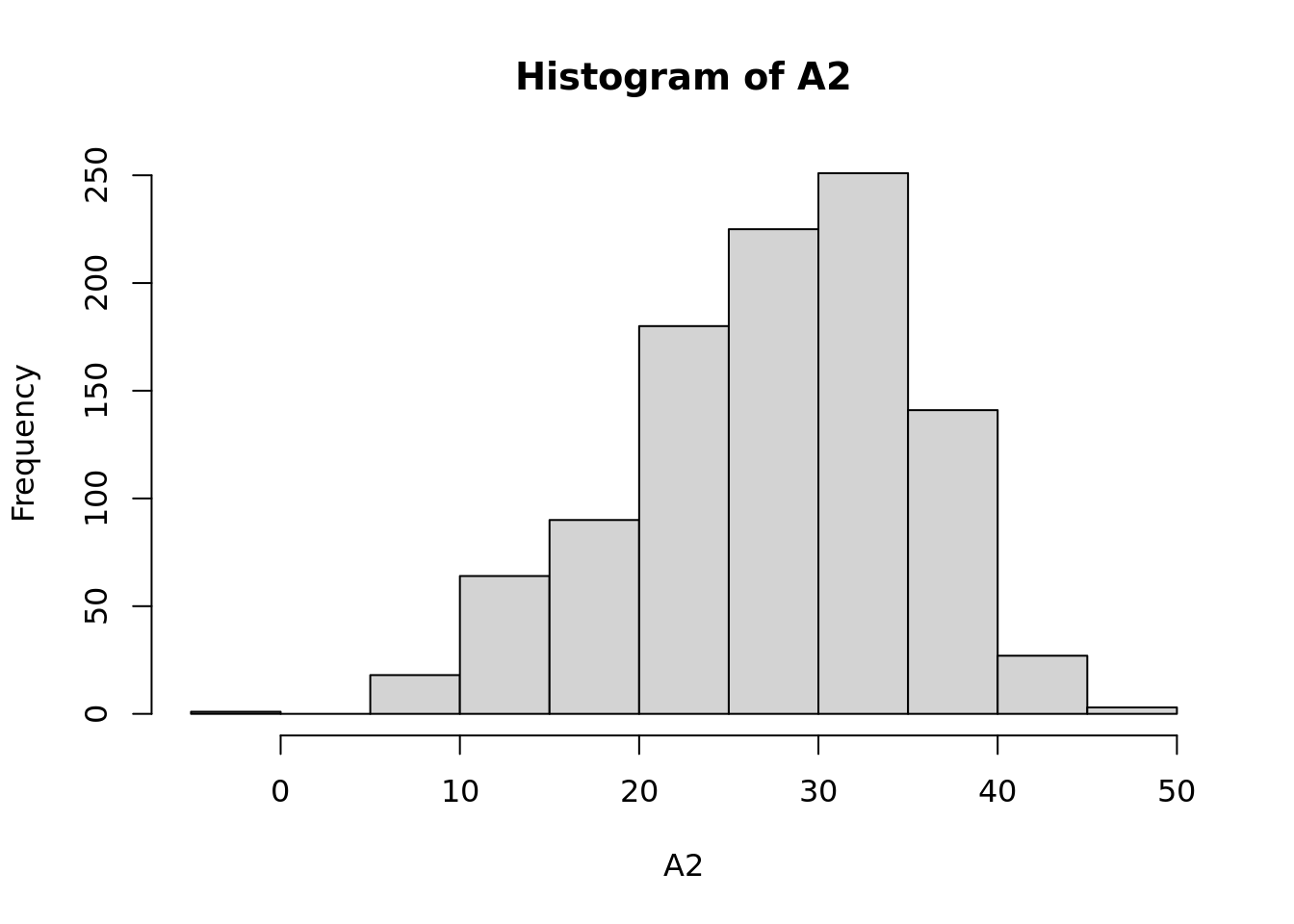Equivalent of sensitivity analysis: (where A2, Gs, Ci are response variables)

pairs(data.frame(A2, Gs, Ci, vmax, Rd, b1, Qp, Tl, RH), pch = '.')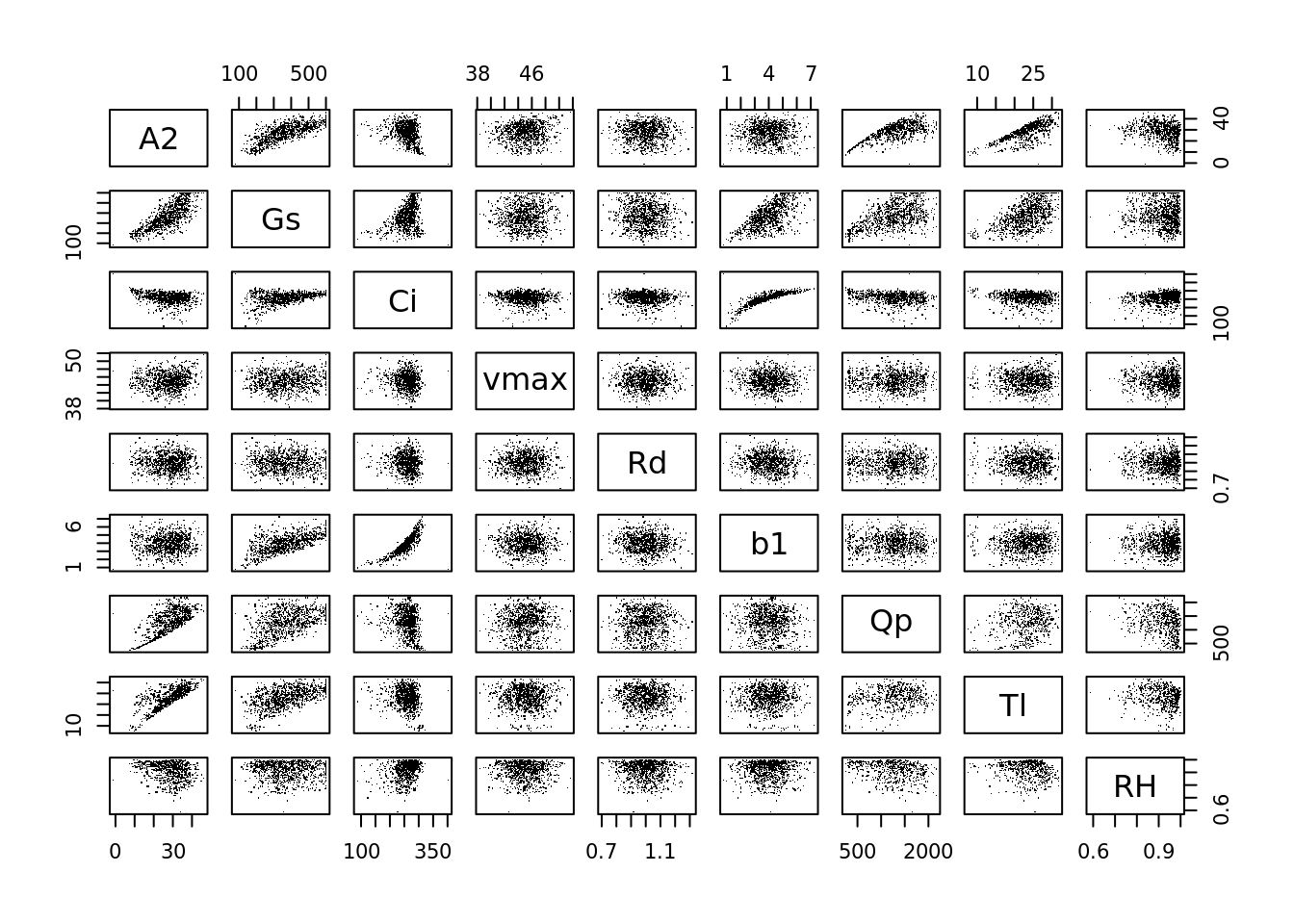The lm is pretty much a sensitivity analysis: what is the slope of the effect of inputs on the output of the model.

summary(lm(A2 ~ vmax + Rd + b1 + Qp + Tl + RH))
##
## Call:
## lm(formula = A2 ~ vmax + Rd + b1 + Qp + Tl + RH)
##
## Residuals:
##    Min     1Q Median     3Q    Max
## -35.33  -1.35   0.71   1.61   6.75
##
## Coefficients:
##              Estimate Std. Error t value Pr(>|t|)
## (Intercept) -2.61e+01   2.42e+00  -10.78  < 2e-16 ***
## vmax         2.94e-01   3.94e-02    7.47  1.8e-13 ***
## Rd           5.76e-02   8.29e-01    0.07     0.94
## b1           3.67e-01   7.75e-02    4.73  2.6e-06 ***
## Qp           1.05e-02   1.93e-04   54.54  < 2e-16 ***
## Tl           1.08e+00   1.86e-02   57.99  < 2e-16 ***
## RH           9.66e-01   1.28e+00    0.76     0.45
## ---
## Signif. codes:
## 0 '***' 0.001 '**' 0.01 '*' 0.05 '.' 0.1 ' ' 1
##
## Residual standard error: 2.56 on 993 degrees of freedom
## Multiple R-squared:  0.895,  Adjusted R-squared:  0.894
## F-statistic: 1.41e+03 on 6 and 993 DF,  p-value: <2e-16

The analysis of variance partitions the variance - how much if the total variance in A2 is contributed by each of the following parameters (recall that the domain for met variables is << the domain for physiological parameters …

What would happen if we used a whole year of meteorological data instead of the one hour of met data that we used?

summary(aov(A2 ~ vmax + Rd + b1 + Qp + Tl + RH))
##              Df Sum Sq Mean Sq F value Pr(>F)
## vmax          1    932     932  142.26 <2e-16 ***
## Rd            1     21      21    3.21  0.074 .
## b1            1     33      33    5.01  0.025 *
## Qp            1  32153   32153 4906.97 <2e-16 ***
## Tl            1  22380   22380 3415.44 <2e-16 ***
## RH            1      4       4    0.57  0.450
## Residuals   993   6507       7
## ---
## Signif. codes:
## 0 '***' 0.001 '**' 0.01 '*' 0.05 '.' 0.1 ' ' 1
tidy(aov(Gs ~ vmax + Rd + b1 + Qp + Tl + RH))

tidy(aov(Ci ~ vmax + Rd + b1 + Qp + Tl + RH))

### 4.3.5 References

Taylor, K.E., R.J. Stouffer, G.A. Meehl: An Overview of CMIP5 and the experiment design.” Bull. Amer. Meteor. Soc., 93, 485-498, doi:10.1175/BAMS-D-11-00094.1, 2012. http://journals.ametsoc.org/doi/pdf/10.1175/BAMS-D-11-00094.1

Humphries S and Long SP (1995) WIMOVAC - a software package for modeling the dynamics of the plant leaf and canopy photosynthesis. Computer Applications in the Bioscience 11(4):361-371.

Miguez FE, Zhu XG, Humphries S, Bollero GA, Long SP (2009) A semimechanistic model predicting the growth and production of the bioenergy crop Miscanthus × giganteus: description, parameterization and validation. Global Change Biology Bioenergy 1: 282-296.

Wang D, Jaiswal D, Lebauer DS, Wertin TM, Bollero GA, Leakey ADB, Long SP (2015) A physiological and biophysical model of coppice willow (Salix spp.) production yields for the contiguous USA in current and future climate scenarios. Plant, cell & environment, 38(9), 1850-1865.

Thornton, M.M., R. Shrestha, Y. Wei, P.E. Thornton, S. Kao, and B.E. Wilson. 2020. Daymet: Daily Surface Weather Data on a 1-km Grid for North America, Version 4. ORNL DAAC, Oak Ridge, Tennessee, USA. https://doi.org/10.3334/ORNLDAAC/1840

Daymet: Daily Surface Weather Data on a 1-km Grid for North America, Version 4 https://doi.org/10.3334/ORNLDAAC/1840